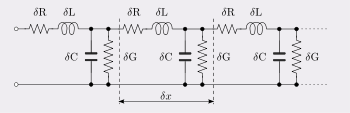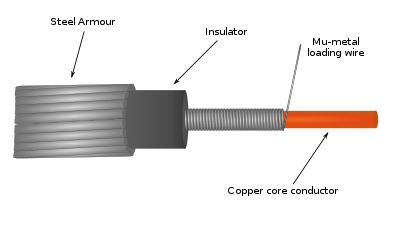# Heaviside condition

﻿
Heaviside condition

The Heaviside condition, due to Oliver Heaviside (1850–1925), is the condition an electrical transmission line must meet in order for there to be no distortion of a transmitted signal. Also known as the distortionless condition, it can be used to improve the performance of a transmission line by adding loading to the cable.

## The conditionHeaviside's model of a transmission line.

A transmission line can be represented as a distributed element model of its primary line constants as shown in the figure. The primary constants are the electrical properties of the cable per unit length and are: capacitance C (in farads per meter), inductance L (in henries per meter), series resistance R (in ohms per meter), and shunt conductance G (in siemens per meter). The series resistance and shunt conductivity cause losses in the line; for an ideal transmission line,$R=G=0$.

The Heaviside condition is satisfied when$\frac{G}{C} = \frac{R}{L}.$

This condition is for no distortion, but not for no loss.

## Background

A signal on a transmission line can become distorted even if the line constants, and the resulting transmission function, are all perfectly linear. This happens in two ways: firstly, the attenuation of the line can vary with frequency which results in a change to the shape of a pulse transmitted down the line. Secondly, and usually more problematically, distortion is caused by a frequency dependence on phase velocity of the transmitted signal frequency components. If different frequency components of the signal are transmitted at different velocities the signal becomes "smeared out" in space and time, a form of distortion called dispersion.

This was a major problem on the first transatlantic telegraph cable and led to the theory of the causes of dispersion being investigated first by Lord Kelvin and then by Heaviside who discovered how it could be countered. Dispersion of telegraph pulses, if severe enough, will cause them to overlap with adjacent pulses, causing what is now called intersymbol interference. To prevent intersymbol interference it was necessary to reduce the transmission speed of the transatlantic telegraph cable to the equivalent of 115 baud. This is an exceptionally slow data transmission rate, even for human operators who had great difficulty operating a morse key that slowly.

For voice circuits (telephone) the frequency response distortion is usually more important than dispersion whereas digital signals are highly susceptible to dispersion distortion. For any kind of analogue image transmission such as video or facsimile both kinds of distortion need to be eliminated.

## Derivation

The transmission function of a transmission line is defined in terms of its input and output voltages when correctly terminated (that is, with no reflections) as$\frac{V_\mathrm{in}}{V_\mathrm{out}} = e^{\gamma x}$

where x represents distance from the transmitter in meters and$\gamma = \alpha +j \beta \,$

are the secondary line constants, α being the attenuation in nepers per metre and β being the phase change constant in radians per metre. For no distortion, α is required to be constant with angular frequency ω, while β must be proportional to ω. This requirement for proportionality to frequency is due to the relationship between the velocity, v, and phase constant, β being given by,$v = \frac{\omega}{\beta}$

and the requirement that phase velocity, v, be constant at all frequencies.

The relationship between the primary and secondary line constants is given by$\gamma^2 = (\alpha +j \beta)^2 = (R+j \omega L)(G + j \omega C)\,$

which has to be of the form$(A+j\omega B)^2$ in order to meet the distortionless condition. The only way this can be so is if$(R+j \omega L)$ and$(G + j \omega C)$ differ by no more than a constant factor. Since both have a real and imaginary part, the real and imaginary parts must independently be related by the same factor, so that;$\frac {R}{G} = \frac {j \omega L}{j \omega C}$

and the Heaviside condition is proved.

### Line characteristics

The secondary constants of a line meeting the Heaviside condition are consequently, in terms of the primary constants:

Attenuation,$\alpha = \sqrt {RG}$  nepers/metre

Phase change constant,$\beta = \omega \sqrt {LC}$  radians/metre

Phase velocity,$v = \frac {1}{\sqrt {LC}}$  metres/second

### Characteristic impedance

The characteristic impedance of a lossy transmission line is given by$Z_0=\sqrt{\frac{R+j\omega L}{G+j\omega C}}$

In general, it is not possible to match this transmission line at all frequencies because the square root causes the expression to be irrational and it consequently cannot be realised exactly with a network of discrete elements. However, for a line which meets the Heaviside condition, there is a common factor in the fraction which cancels out the frequency dependent terms leaving,$Z_0=\sqrt{\frac{L}{C}},$

which is a real number, and independent of frequency. The line can therefore be matched with just a resistor at either end. This expression for$Z_0 = \sqrt{L/C}$ is the same as for a lossless line ($R = 0,\ G = 0$) with the same L and C, although the attenuation (due to R and G) is of course still present.

## Practical useA real line, especially one using modern synthetic insulators, will have a G that is very low and will usually not come anywhere close to meeting the Heaviside condition. The normal situation is that$\frac{G}{C} \ll \frac{R}{L}.$

To make a line meet the Heaviside condition one of the four primary constants needs to be adjusted and the question is which one. G could be increased, but this is highly undesirable since increasing G will increase the loss. Decreasing R is sending the loss in the right direction, but this is still not usually a satisfactory solution. R must be decreased by a large fraction and to do this the conductor cross-sections must be increased dramatically. This not only makes the cable much more bulky but also adds significantly to the amount of copper (or other metal) being used and hence the cost. Decreasing the capacitance also makes the cable more bulky (since the insulation must now be thicker) but is not so costly as increasing the copper content. This leaves increasing L which is the usual solution adopted.

The required increase in L is achieved by loading the cable with a metal with high magnetic permeability. It is also possible to load a cable of conventional construction by adding discrete loading coils at regular intervals. This is not identical to a distributed loading, the difference being that with loading coils there is distortionless transmission up to a definite cut-off frequency beyond which the attenuation increases rapidly.

Loading cables to meet the Heaviside condition is no longer a common practice. Instead, regularly spaced digital repeaters are now placed in long lines to maintain the desired shape and duration of pulses for long-distance transmission.

Wikimedia Foundation. 2010.

### Look at other dictionaries:

• Heaviside (disambiguation) — Heaviside usually refers to the mathematician Oliver Heaviside, but it can refer to:* Heaviside step function, named in honor of him. * Heaviside condition, named in honor of him. * Heaviside layer, or Kennelly Heaviside layer, named in honor of… …   Wikipedia

• Oliver Heaviside — Heaviside redirects here. For other uses, see Heaviside (disambiguation). Oliver Heaviside Portrait by Francis Edwin Hodge …   Wikipedia

• Loading coil — In electronics, a loading coil or load coil is a coil (inductor) that does not provide coupling to any other circuit, but is inserted in a circuit to increase its inductance. The need was discovered by Oliver Heaviside in studying the… …   Wikipedia

• George Ashley Campbell — Infobox Scientist name = George Ashley Campbell caption = birth date = birth date|1870|11|27 birth place = Hastings, Minnesota death date = death date and age|1954|11|10|1870|11|27 death place = residence = United States nationality =American… …   Wikipedia

• Transmission line — This article is about the radio frequency transmission line. For the power transmission line, see electric power transmission. In communications and electronic engineering, a transmission line is a specialized cable designed to carry alternating… …   Wikipedia

• Carl Emil Krarup — Infobox Scientist box width = name = Carl Emil Krarup image size = caption = birth date = 1872 birth place = Copenhagen, Denmark death date = 1909 death place = residence = Copenhagen citizenship = nationality = Danish ethnicity = fields =… …   Wikipedia

• Propagation constant — The propagation constant of an electromagnetic wave is a measure of the change undergone by the amplitude of the wave as it propagates in a given direction. The quantity being measured can be the voltage or current in a circuit or a field vector… …   Wikipedia

• Histoire de la relativité restreinte — L’histoire de la relativité restreinte décrit le développement de propositions et constatations empiriques et conceptuelles, au sein de la physique théorique, qui ont permis d’aboutir à une nouvelle compréhension de l’espace et du temps. Cette… …   Wikipédia en Français

• Step response — The step response of a system in a given initial state consists of the time evolution of its outputs when its control inputs are Heaviside step functions. In electronic engineering and control theory, step response is the time behaviour of the… …   Wikipedia

• Dirac delta function — Schematic representation of the Dirac delta function by a line surmounted by an arrow. The height of the arrow is usually used to specify the value of any multiplicative constant, which will give the area under the function. The other convention… …   Wikipedia